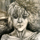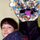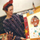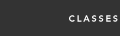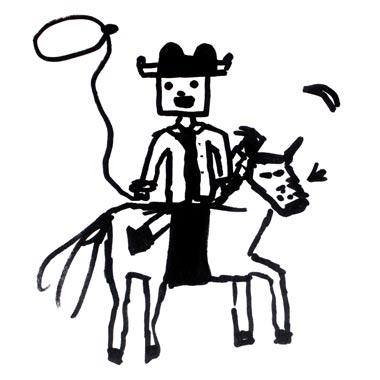B L A C K  &  W H I T EA R T  E N E R G Y  W O R K S H O P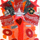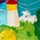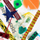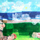A R T S  A T  T H E  C E N T E R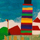P O R T R A I T S